Next: Moment of Inertia Tensor Up: Rigid Body Rotation Previous: Introduction

# Fundamental Equations

We can think of a rigid body as a collection of a large number of small mass elements which all maintain a fixed spatial relationship with respect to one another. Let there be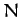elements, and let the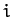th element be of mass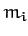, and instantaneous position vector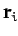. The equation of motion of theth element is written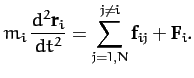(454)

Here,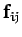is the internal force exerted on theth element by the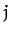th element, and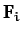the external force acting on theth element. The internal forcesrepresent the stresses which develop within the body in order to ensure that its various elements maintain a constant spatial relationship with respect to one another. Of course,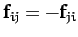, by Newton's third law. The external forces represent forces which originate outside the body.

Repeating the analysis of Section 2.7, we can sum Equation (454) over all mass elements to obtain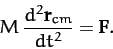(455)

Here,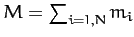is the total mass,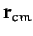the position vector of the center of mass [see Equation (27)], and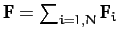the total external force. It can be seen that the center of mass of a rigid body moves under the action of the external forces like a point particle whose mass is identical with that of the body.

Again repeating the analysis of Section 2.7, we can sum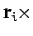Equation (454) over all mass elements to obtain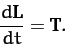(456)

Here,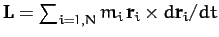is the total angular momentum of the body (about the origin), and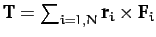the total external torque (about the origin). Note that the above equation is only valid if the internal forces are central in nature. However, this is not a particularly onerous constraint. Equation (456) describes how the angular momentum of a rigid body evolves in time under the action of the external torques.

In the following, we shall only consider the rotational motion of rigid bodies, since their translational motion is similar to that of point particles [see Equation (455)], and, therefore, fairly straightforward in nature.Next: Moment of Inertia Tensor Up: Rigid Body Rotation Previous: Introduction
Richard Fitzpatrick 2011-03-31